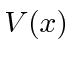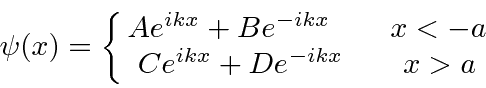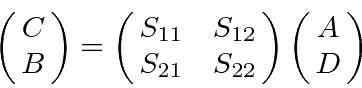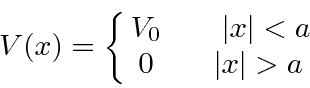## Homework 3

1. A general one dimensional scattering problem could be characterized by an (arbitrary) potentialwhich is localized by the requirement thatfor. Assume that the wave-function isRelating the outgoing'' waves to the incoming'' waves by the matrix equationshow thatUse this to show that thematrix is unitary.

2. Calculate thematrix for the potentialand show that the above conditions are satisfied.

3. The odd bound state solution to the potential well problem bears many similarities to the zero angular momentum solution to the 3D spherical potential well. Assume the range of the potential iscm, the binding energy is -2.9 MeV, and the mass of the particle is 940 MeV. Find the depth of the potential in MeV. (The equation to solve is transcendental.)

4. Find the three lowest energy wave-functions for the harmonic oscillator.

5. Assume the potential for particle bound inside a nucleus is given byand that the particle has massand energy. Estimate the lifetime of the particle inside this potential.

Jim Branson 2013-04-22